Mass

Mass: How much matter is in an object.

We measure mass by weighing, but weight and mass are not really the same thing.

Mass can be measured using the following units:

• Gram (g)
• Kilogram (kg)
• Tonne (t)

Grams are the smallest, Tonnes are the biggest.

To work out conversions involving units of mass we use the relationships.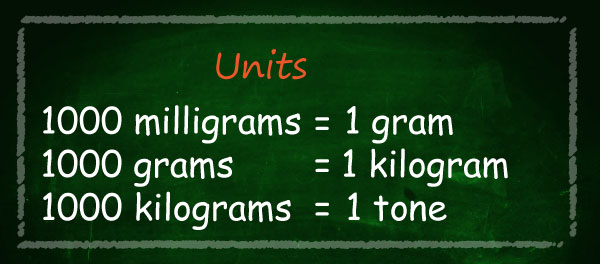•  1 000 g = 1 kg
• 1 000 kg = 1t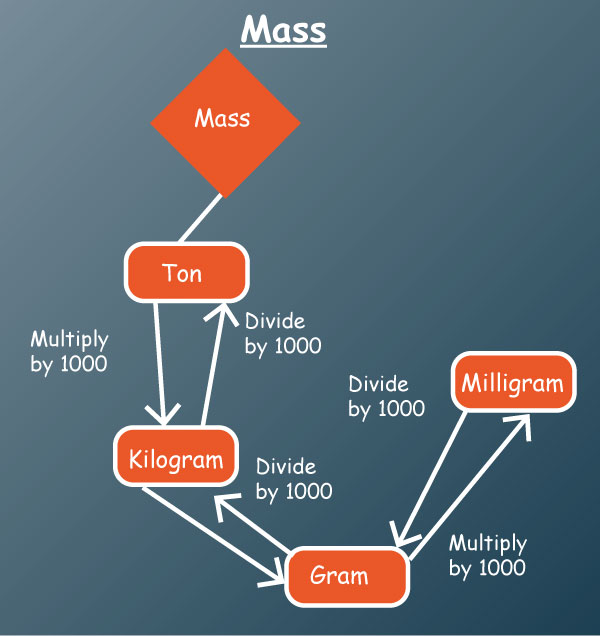#### Example

A supermarket received a load of tea of mass 1.2 t, the tea was later packed into equal number of 500 g and 150 g packets.

Calculate:

1. Total number of packets and amount of tea that remained
2. Amount of tea packed in 150 kg packets.

#### Solution

•   1t = 1 000 kg = 1 000 000 g

1.2t = 1.2 X 1 000 000 g

= 1 200 000 g

• One 500 g and one 150 g packets together

have a mass of 500 g + 150 g = 650 g

Number of packets of each category of packets is

1 200 000 / 650

= 1 846 packets remainder 100 g

Total number of packets

= 2 X 1 846 = 3 692 packets

Amount of tea left over is 100 g

•   Mass of one packet = 150 g

Mass of 1 846 packets = 1 846 X 150 g

= 276.9 kg

A paperclip weighs about 1 gram.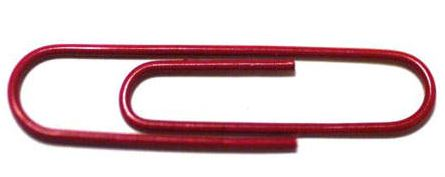• Hold one small paperclip in your hand.

Does that weigh a lot?

No. A gram is very light.

That is why you often see things measured in hundreds of grams.

Grams are often written as g (for short), so "300 g" means "300 grams".

A loaf of bread weighs about 700 g (for a nice sized loaf)

Once you have 1,000 grams, you have 1 kilogram.

• 1 kilogram = 1,000 grams

A dictionary has a mass of about one kilogram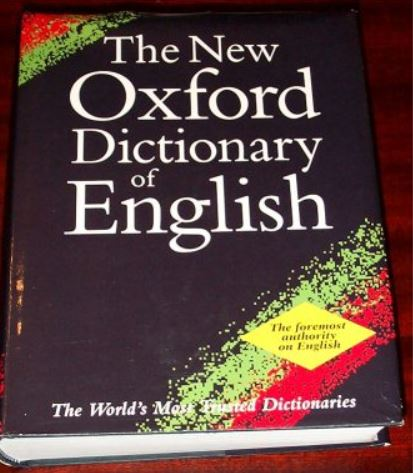Kilograms are great for measuring things that can be lifted by people    (sometimes very strong people are needed of course!)

Kilograms are often written as kg (that is a "k" for "kilo" and a "g" for "gram), so "10 kg" means "10 kilograms".

When you weigh yourself on a scale, you would use kilograms.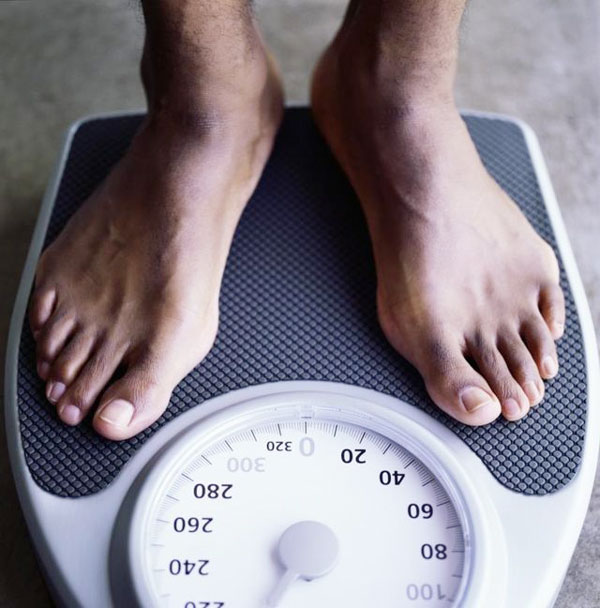How much do you weigh?

But when it comes to things that are very heavy, we need to use the tonne.

Once you have 1,000 kilograms, you will have 1 tonne.

• 1 tonne = 1,000 kilograms

Tonnes (also called Metric Tons) are used to measure things that are very heavy.

Things like cars, trucks and large cargo boxes are weighed using the tonne.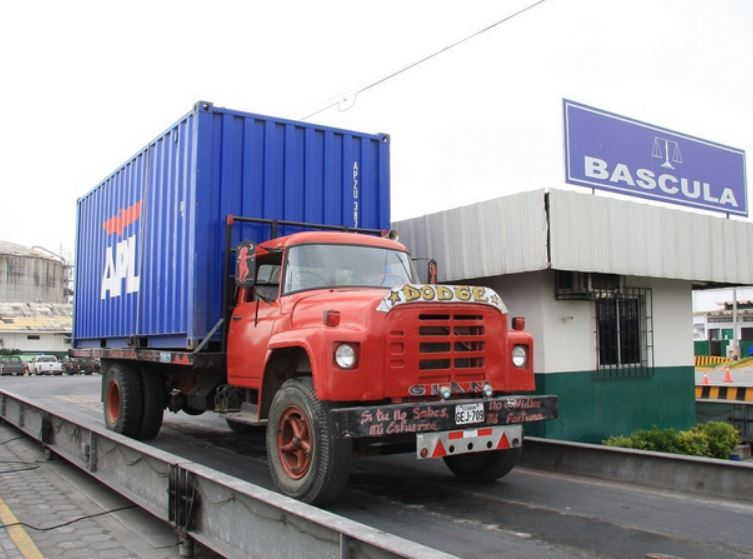This lorry weighs about 5 tonnes.

Tonnes are often written as t (for short), so "5 t" means "5 tonnes".

• Q: Aren't "weight" and "mass" the same?

A: Not really.

• An object has mass (say 100 kg).

This makes it heavy enough to show a weight of "100 kg".

• Gravity causes Weight

An objects weight is how hard gravity is pulling on it.

• We think the weight is the same everywhere ... because we all live on the surface of the planet Earth!

But in orbit it would not push on the scales at all.

• The scales would show 0 kg ... but the mass is still 100 kgAn object's mass doesn't change (unless you remove some), but its weight can change.

So Why Do People Say Weight instead of Mass?

People often use "weight" to mean "mass", and vice versa.

Because gravity is pretty much the same everywhere on Earth, we don't notice a difference

• But remember .. they do not mean the same thing, and they can have different measurements.

Here are some conditions where the Weight might change:

• in space (can be weightless!)
• on the moon (a 100 kg mass would weigh 16.6 kg)
• you can even get very slight differences in weight in different locations on earth.

## KCPE Topical Questions

Class 8

### Mass

1. A container with shoe polish weighs 50 g. What is the weight in tonnes of 600 such containers?

A. 0.3      B. 3      C. 0.03         D. 300

2. A carton containing books weighs 2 kg, The weight of the empty carton is 0.5 kg. What is the total weight of the books contained in 100 such cartons?

A. 50 kg       B. 150 kg      C. 200 kg       D. 250 kg

3. An empty carton weighs 1 kg. Ten packets of soap powder, each weighing 500 g, are packed in one carton. What is the weight of fifty such cartons full of soap?

A. 50 kg      B. 75 kg      C. 250 kg        D. 300 kg

4. A certain powder is packed in 1250 g packets. How many such packets will weigh one tonne?

A. 8000      B. 800      C. 80      D. 8

5. Five hundred kilograms of ta were packed in 500 g packets. How many packets were obtained?

A. 10      B. 100     C. 1000      D. 10000

6. A lorry carried 50 cartons of books and 200 cartons of milk. Each carton of books weighed 40 kilograms and each carton of milk weighed 5 kilograms. What was the total weight, in tonnes, of milk and books?

A. 30 tonnes     B. 1.25 tonnes    C. 3 tonnes     D. 8.25 tonnes